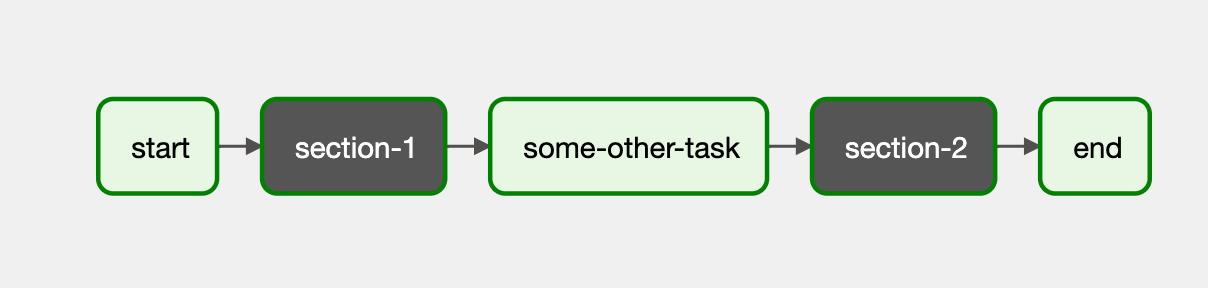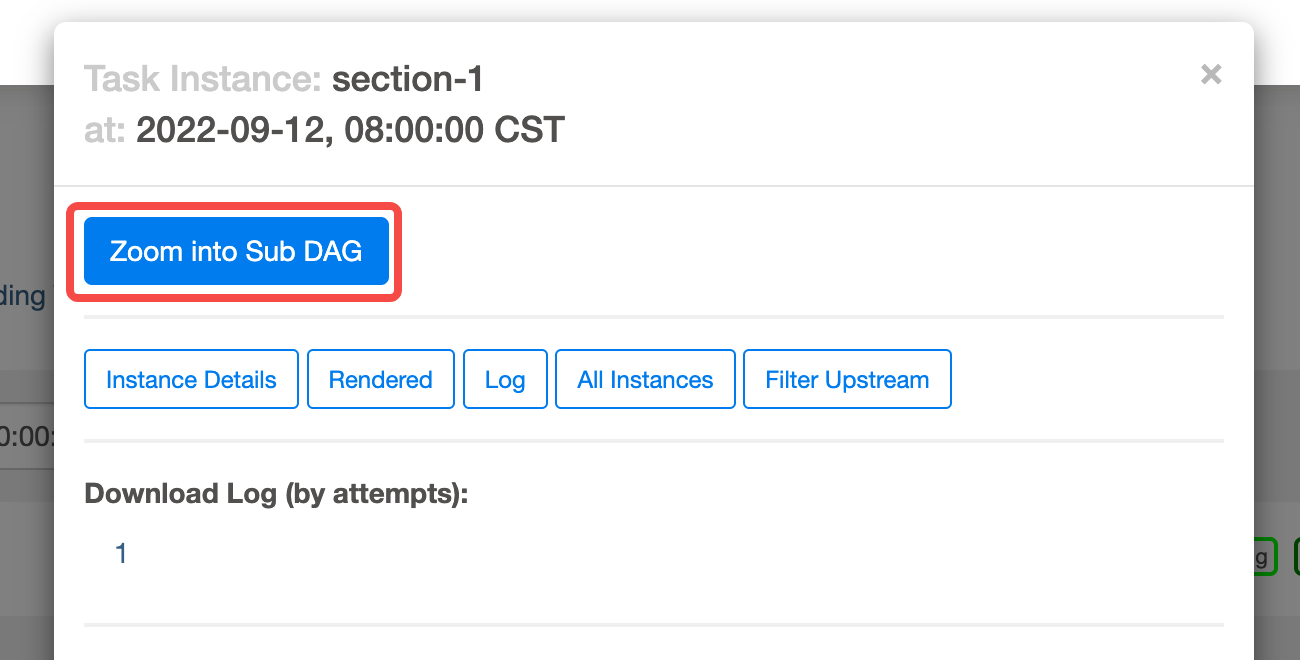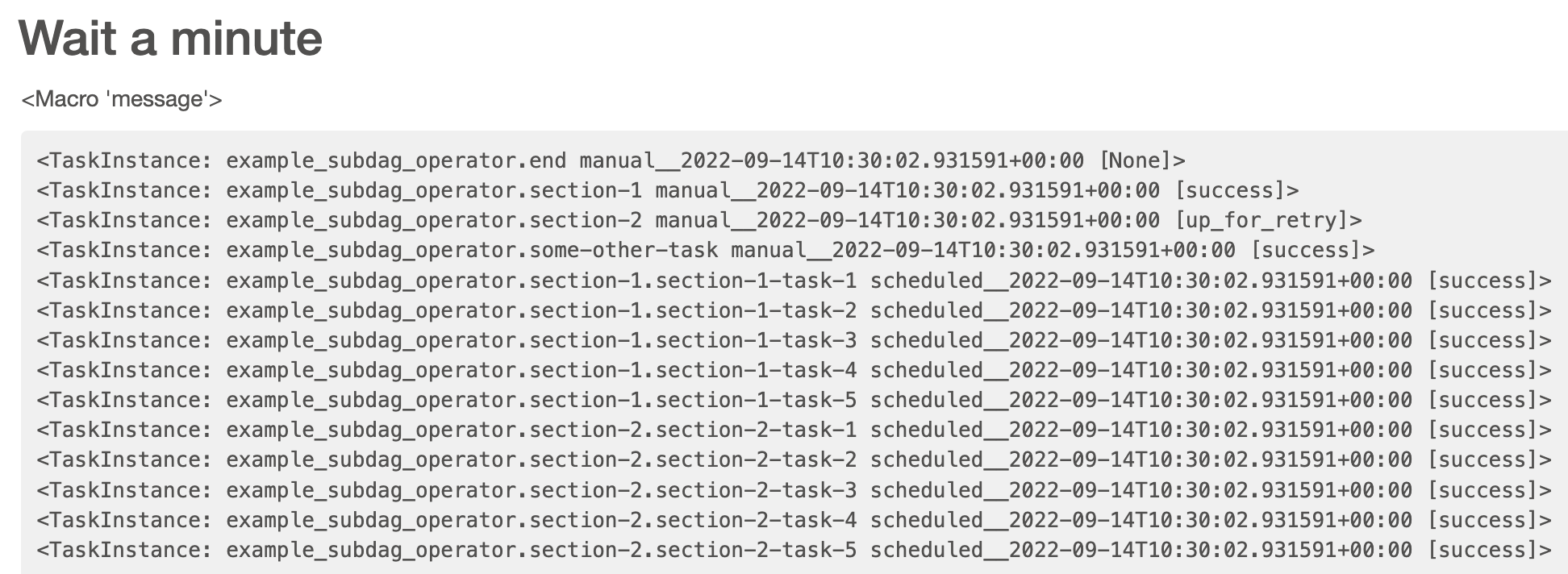You need to enable JavaScript to run this app.

# 高阶使用

## 1 DAG 控制流

### 1.1 Branching Operator

Airflow 提供了一个`BranchPythonOperator`，它接受一个`python_callable`，要求该函数返回一个`task_id`（或者是一个`task_id`的列表），用以决定当前流程往后执行时应该走向某个或者某些特定的分支。

``````from datetime import datetime

from airflow.decorators import dag
from airflow.operators.dummy import DummyOperator
from airflow.operators.python import BranchPythonOperator

@dag(schedule_interval=None, start_date=datetime(2022, 9, 14), catchup=False, tags=['example'])
def demo_dag():

return "branch_a"

branching = BranchPythonOperator(
)

start >> branching
branching >> join

dag = demo_dag()
``````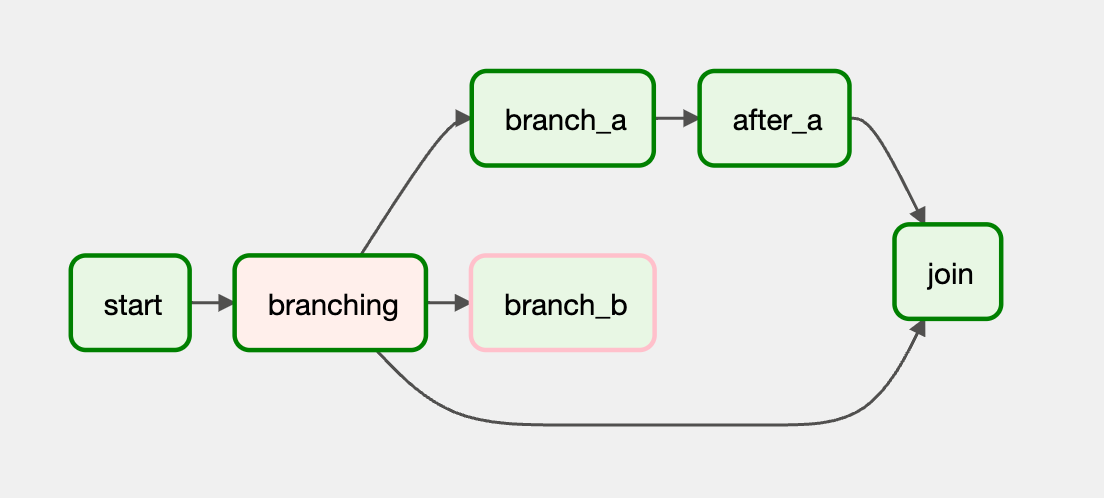### 1.3 Only Latest

`LatestOnlyOperator`在当前的 DAG Run 不是最新的时候，会自动跳过其所有下游。

``````import datetime

from airflow import DAG
from airflow.operators.dummy import DummyOperator
from airflow.operators.latest_only import LatestOnlyOperator
from airflow.utils.trigger_rule import TriggerRule

with DAG(
dag_id='latest_only_with_trigger',
schedule_interval=datetime.timedelta(hours=6),
start_date=datetime.datetime(2022, 9, 1),
catchup=True,
tags=['example3'],
) as dag:

``````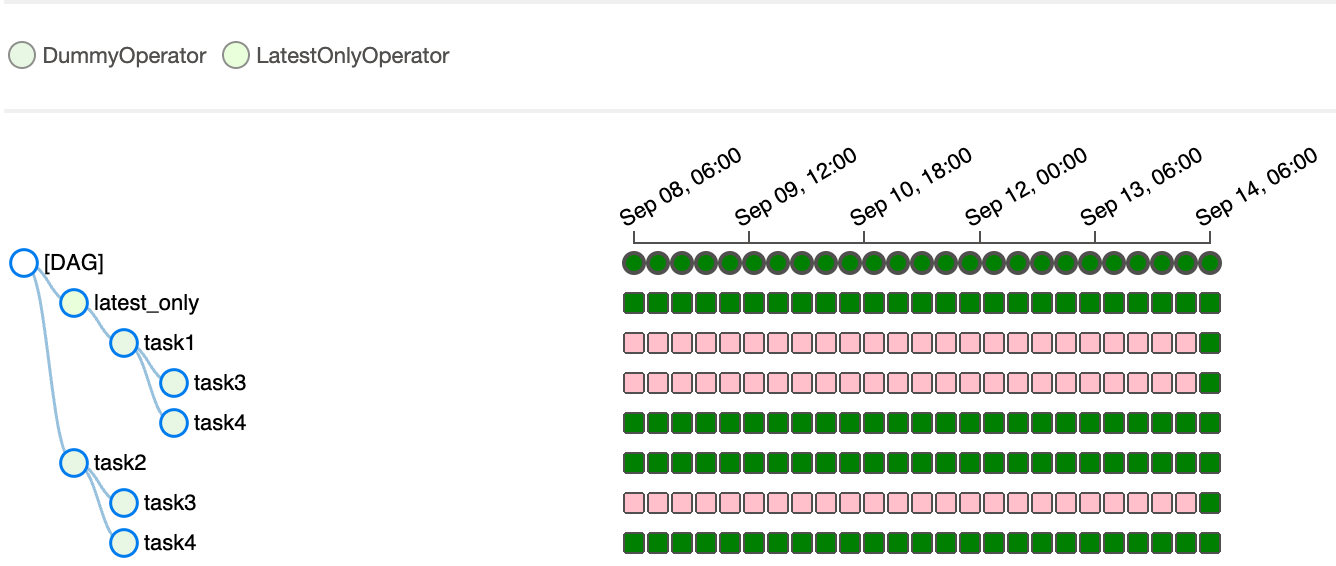### 1.4 Trigger Rules

• all_success：缺省值，需要全部上游任务都成功。

• all_failed：需要全部上游任务处于`failed`或者`upstream_failed`状态。

• all_done：所有上游都完成各自的执行。

• one_failed：至少有一个上游任务失败，不需要等待所有上游结束。

• one_success：至少有一个上游任务成功，不需要等待所有上游结束。

• none_failed：所有上游任务要么成功，要么被跳过。

• none_failed_min_one_success：所有上游任务至少有一个成功，且没有失败的情况。

• none_skipped：没有任何上游任务被跳过。

• always：没有任何上游依赖，处于随时可以被调度执行的状态。

``````from datetime import datetime

from airflow.decorators import dag
from airflow.operators.dummy import DummyOperator
from airflow.operators.python import BranchPythonOperator

@dag(schedule_interval=None, start_date=datetime(2022, 9, 14), catchup=False, tags=['example'])
def demo_dag_c():

return "branch_a"

branching = BranchPythonOperator(
)

start >> branching
branching >> join

dag = demo_dag_c()
``````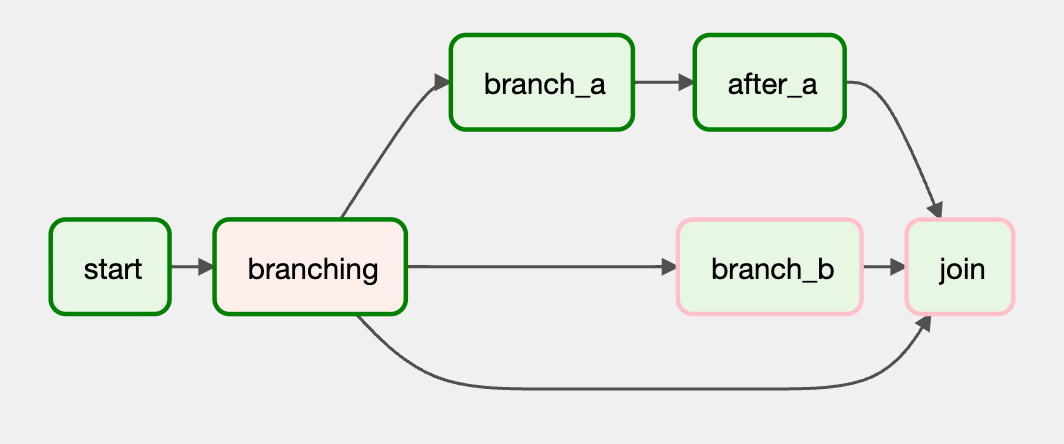``````from datetime import datetime

from airflow.decorators import dag
from airflow.operators.dummy import DummyOperator
from airflow.operators.python import BranchPythonOperator
from airflow.utils.trigger_rule import TriggerRule

@dag(schedule_interval=None, start_date=datetime(2022, 9, 14), catchup=False, tags=['example2'])
def demo_dag_d():

return "branch_a"

branching = BranchPythonOperator(
)

start >> branching
branching >> join

dag = demo_dag_d()
``````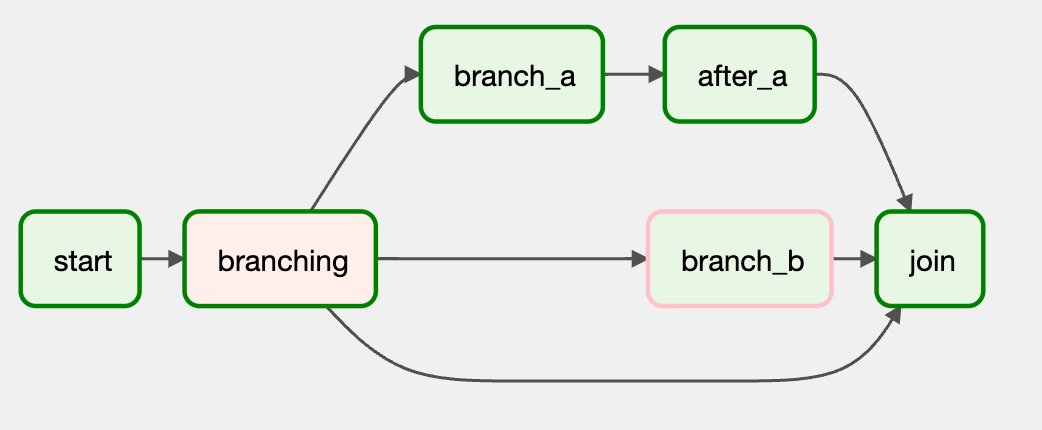## 2 DAG 可视化定义

### 2.1 任务分组

``````with TaskGroup("group1") as group1:

``````

``````with DAG(dag_id='dag1', default_args={'scope': 'DAG'}) as dag:

with TaskGroup("group1", default_args={'scope': 'group1'}) as group1:

``````

### 2.2 边的标签化

``````from airflow.utils.edgemodifier import Label

``````

``````from airflow.utils.edgemodifier import Label

``````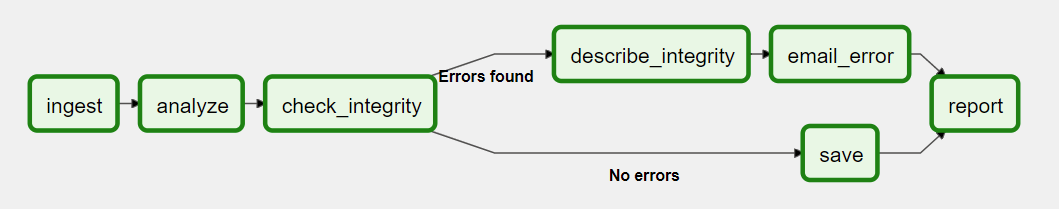## 3 SubDAGs

``````from datetime import datetime

from airflow import DAG
from airflow.operators.dummy import DummyOperator

def subdag(parent_dag_name, child_dag_name, args):
"""
Generate a DAG to be used as a subdag.

:param str parent_dag_name: Id of the parent DAG
:param str child_dag_name: Id of the child DAG
:param dict args: Default arguments to provide to the subdag
:return: DAG to use as a subdag
:rtype: airflow.models.DAG
"""
dag_subdag = DAG(
dag_id=f'{parent_dag_name}.{child_dag_name}',
default_args=args,
start_date=datetime(2021, 1, 1),
catchup=False,
schedule_interval="@daily",
)

for i in range(5):
DummyOperator(
default_args=args,
dag=dag_subdag,
)

return dag_subdag
``````

``````from airflow import DAG
from airflow.example_dags.subdags.subdag import subdag
from airflow.operators.dummy import DummyOperator
from airflow.operators.subdag import SubDagOperator
from airflow.utils.dates import days_ago

DAG_NAME = 'example_subdag_operator'

args = {
'owner': 'airflow',
}

with DAG(
dag_id=DAG_NAME, default_args=args, start_date=days_ago(2), schedule_interval="@once", tags=['example']
) as dag:

start = DummyOperator(
)

section_1 = SubDagOperator(
subdag=subdag(DAG_NAME, 'section-1', args),
)

)

section_2 = SubDagOperator(
subdag=subdag(DAG_NAME, 'section-2', args),
)

end = DummyOperator(
)

start >> section_1 >> some_other_task >> section_2 >> end
# [END example_subdag_operator]
``````